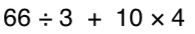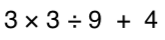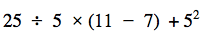Order of Operations
Distributive Property
One-Step Equations
Multi-Step Equations
Exponents
100

Simplify the following expression:100

8(4+2) = ?

48

100
Simplify:

2x + 8x=

10x

100

Solve for X:

X + 8 = 25

x=17

100

3x - 5 = 22

x=9

100

When you see the following expression, what is the big number called and what is the little number on top of it called?

24

200

Simplify the following expression:200

5(x - 3)

5x - 15

200

Simplify:

4x + 2x -8x

-2x

200

Solve for X:

X-3=10

X=13

200

4x - 7 = -31

x = -6

200

How can you rewrite 9in expanded form?

9 x 9

300

3 + 42 * (3 + 2)

83

300

7 + 9(2x - 3)

18x - 20

300
Simplify:

-3x + 2x +5 -2

-x + 3

300
Solve for X:

4x=28

X=7

300

(3/5)x - 9 = 12

x = 35

300

How can you rewrite the following in exponential form?

7 x 7 x 7 x 7 x 7

75

40045

400

-3(-x - 10)

3x + 30

400

Simplify:

-4x +2x -6 +3

-2x-3

400

Solve for X:X=40

400

5x - 3 + 7 - 3x = 12

x = 4

400

What is the value of:

34

81

50012

500

x + 10 - 6(x - 3x) + 4

13x + 14

500

Simplify:

-2(x + 4) + 5x

3x - 8

OR   -8 + 3x
500

Solve for X:

2/3 * x = 18

X=27

500

3(2x - 6) = 4(x - 7)

x = -5

500

What is the value of:

23 + 32 - 42

Click to zoom